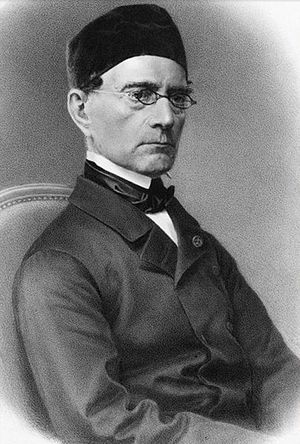Which famous people have you outlived?

Gabriel Lamé

French mathematician

Died when: 74 years 283 days (897 months)
Star Sign: LeoGabriel Lamé (22 July 1795 – 1 May 1870) was a French mathematician who contributed to the theory of partial differential equations by the use of curvilinear coordinates, and the mathematical theory of elasticity (for which linear elasticity and finite strain theory elaborate the mathematical abstractions).